# Edexcel IGCSE Maths 复习笔记 4.9.1 SOHCAHTOA

Edexcel IGCSE Maths 复习笔记 4.9.1 SOHCAHTOA

#### What is SOHCAHTOA?

• SOHCAHTOA is a mnemonic – ie a way to remember the 3 important formulas for sin (sine), cos (cosine), and tan (tangent)
• In a right-angled triangle, label one angle (NOT the right angle!), and label the sides of the triangles as follows: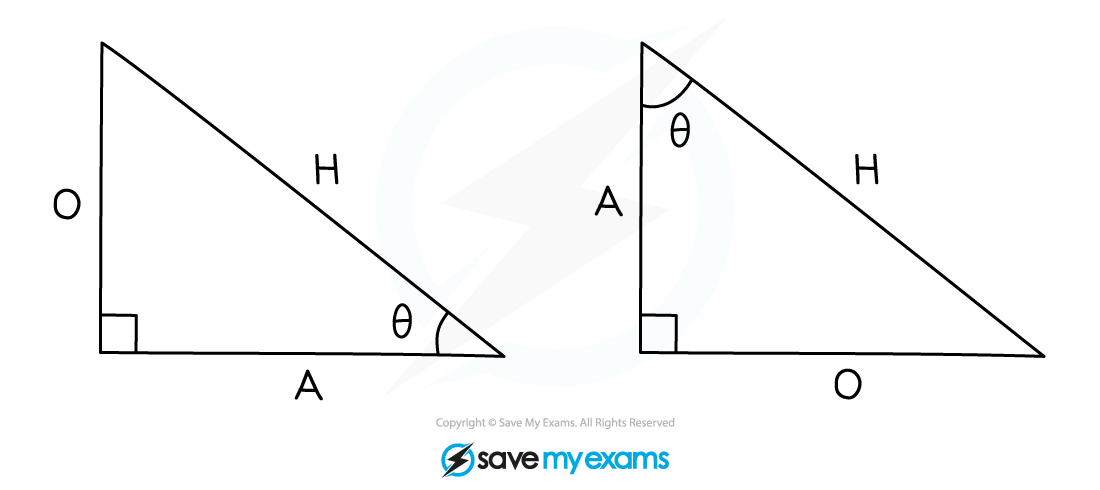• Note that:

θ = Greek letter thetaO = opposite

'H' is always the same, but 'O' and 'A' depend on which angle we're calling θ

• Using those labels, the three SOHCAHTOA equations are:#### Using SOHCAHTOA

1. Label the triangle: the angle you know (or want to know) is 'θ' -- 'O' and 'A' follow from thatChoose SOH, CAH, or TOA

The letters of the sides you're interested in (ie. know or want to know) tell you which to choose

2. To find a side: put and the side you know into the equation from 2, and solve to find the side you don't know
3. To find an angle: use the inverse trig functions on your calculator: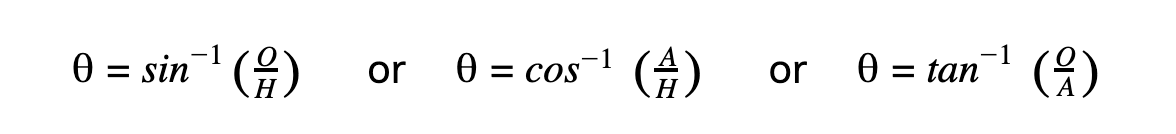#### Exam Tip

SOHCAHTOA (like Pythagoras) can only be used in right-angles triangles – for triangles that are not right-angled, you will need to use the Sine Rule or the Cosine Rule.Also, make sure your calculator is set to measure angles in degrees.

#### Worked Example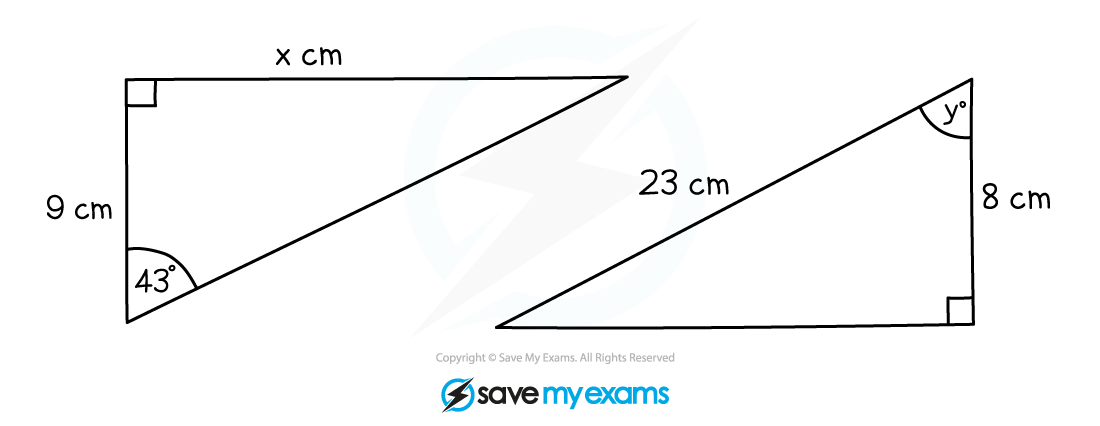#### Worked Example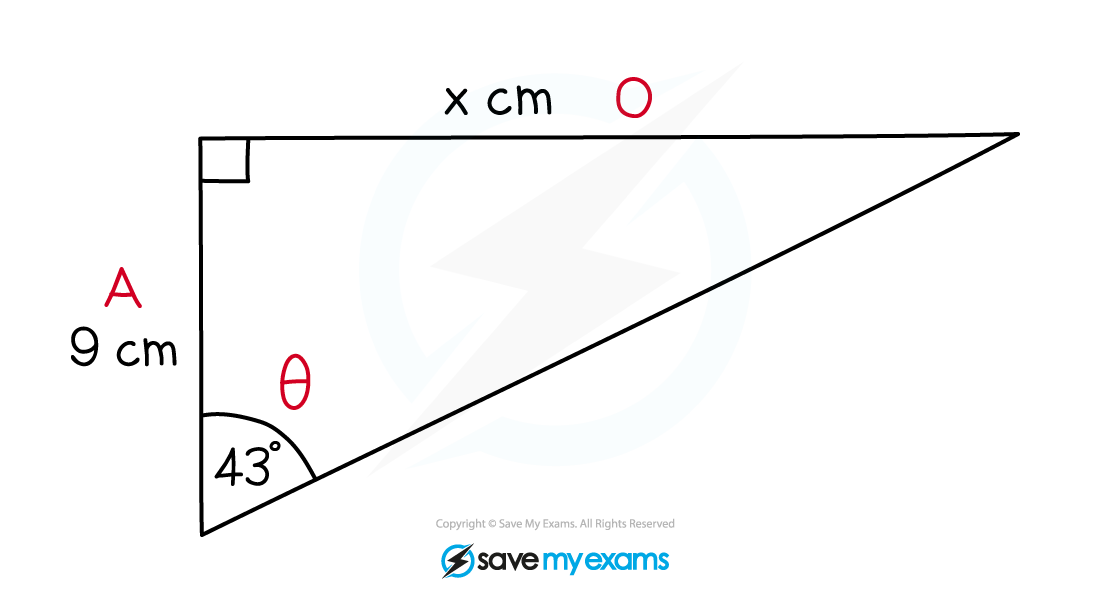#### Worked Example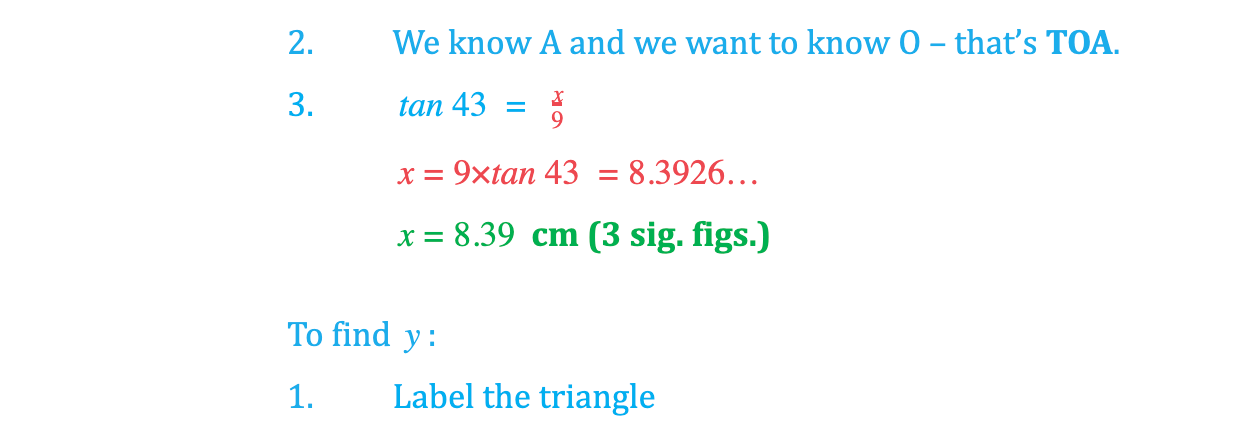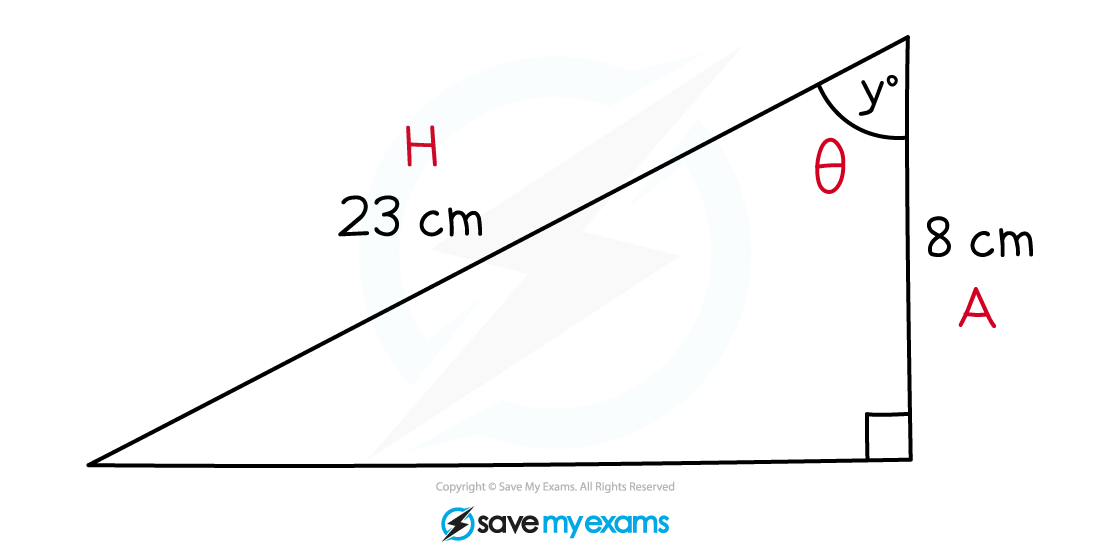#### Worked Example